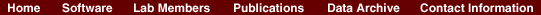Convergence of structure: Taita thrush data.

The series of pictures below shows the values of Q (the estimated population ancestries) at different times in a single run of the Markov chain algorithm. The data are from the thrush example. Each plot shows the current value of Q in a particular iteration (i.e., these are not average values). The final plot shows average results over the course of the run, following a burnin period. The different coloured symbols correspond to individuals collected at different locations.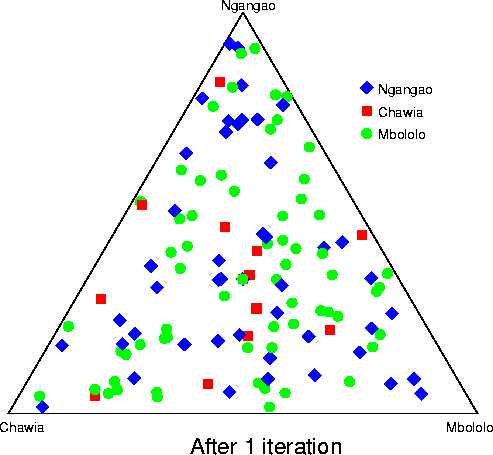This is the initial configuration, in which the q (ancestry vector) for each individual was drawn at random, uniformly on the simplex. Each point shows the q for a single individual. The estimated proportion of ancestry in each population is given by the distance to the edge of the triangle opposite that population's vertex. The labelling of the three vertices differs randomly between independent runs of the Markov chain.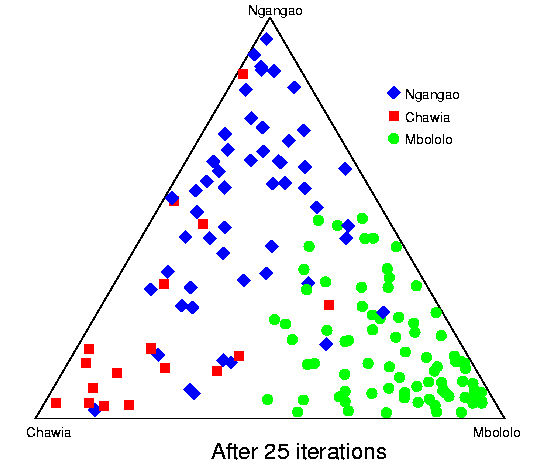After 25 iterations, the points are starting to sort into clusters corresponding to the underlying population structure.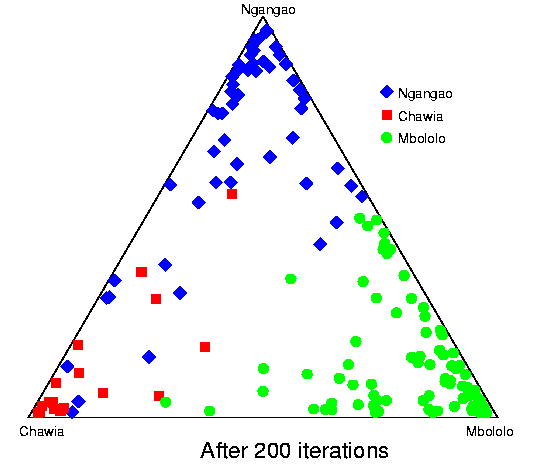Now the estimates are starting to coalesce into the corners. As this happens, the hyperparameter alpha starts to become much smaller than the initial value of 1.0 (the mean posterior estimate is about 0.02). [Recall that the prior for q is Dirichlet(alpha,alpha,alpha).] Meanwhile, though not shown here, the allele frequency estimates for each group start to become more accurate, and this in turn enables better estimation of q.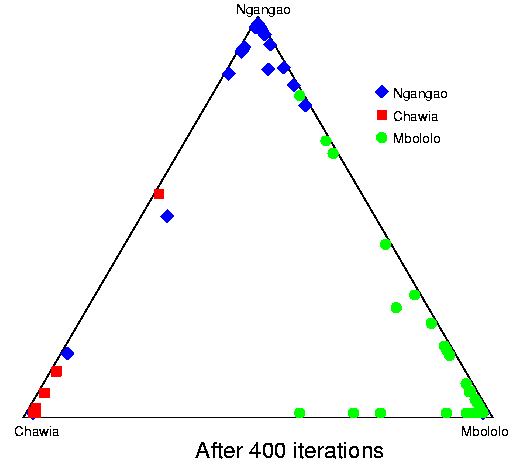The points continue moving towards the edges and corners.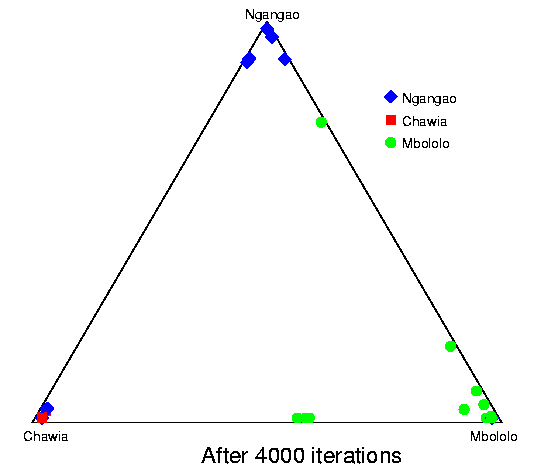After about 2000 iterations, the plots look roughly like this. Most of the 155 points are in the appropriate corners (on top of each other so that they are hard to see), but notice that there are two blue individuals in the Chawia corner, and one in the Mbololo corner. During the course of the Markov chain, some of the points make long excursions along the edges of the simplex even though their average positions may be closer to their "own" vertex of the triangle (next plot). The extent to which the q for a particular individual moves around the simplex gives an indication of how much uncertainty there is in the accuracy of that q. It turns out that for a number of these individuals, the posterior intervals are extremely broad. These results are based on just 7 loci; if we had more data the posterior intervals would be more narrow.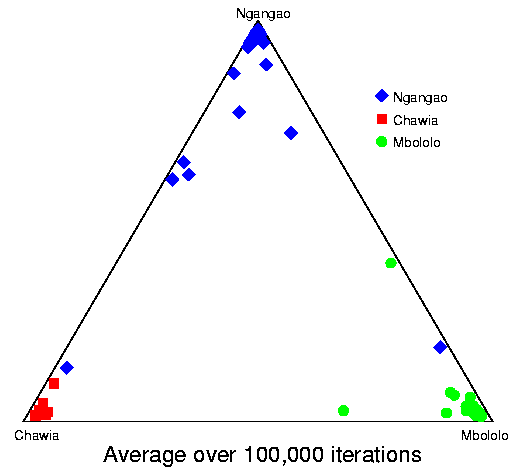Here is a plot of final results for these data. Each point plots the average q over 100,000 iterations, following a burnin of 10,000. All the points in the extreme corners are from the correct populations.